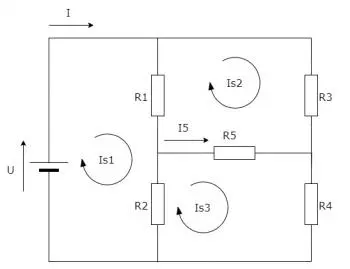# Kirchhoff's Laws: Definition, Examples and ApplicationsElectrical circuits are an essential part of our modern life. From the simplest electronic devices to the most complex power systems, they all depend on the correct understanding and analysis of electrical circuits.

In the 19th century, Gustav Kirchhoff, a German physicist, formulated the laws that bear his name and which have become the basic foundations for circuit analysis: Kirchhoff's laws.

## Kirchhoff's Current Law (node Law):

Kirchhoff's current law, also known as the law of nodes, states that at any node in an electrical circuit, the algebraic sum of the currents entering and leaving that node is equal to zero. In other words, the current flowing into a node is equal to the current leaving that same node.

By applying Kirchhoff's current law, equations can be established that relate the currents in each branch of the circuit and thus determine the global behavior of the system.

## Kirchhoff's Stress Law (mesh Law):

Kirchhoff's voltage law, also known as the mesh law, states that in any closed loop of an electrical circuit, the algebraic sum of the potential differences (voltages) is equal to zero. In other words, the sum of voltage drops and voltage rises in a closed loop is zero.

By applying Kirchhoff's voltage law, equations can be established that relate the voltages in each element of the circuit and allow determining how the voltage is distributed in the system.

## Application of Kirchhoff's Laws:

Kirchhoff's laws are applicable to any electrical circuit, whether in direct current (DC) or alternating current (AC). These laws are especially useful for analyzing complex circuits, where there are multiple sources of current and voltage, as well as a variety of elements such as resistors, capacitors, and inductors.

By applying Kirchhoff's laws, it is possible to establish a system of equations that can be solved to determine the currents and voltages in each component of the circuit. This allows understanding the global behavior of the system and performing precise calculations to design efficient and reliable circuits.

## What Are Kirchhoff's Laws For?

Kirchhoff's laws are fundamental tools for the analysis and design of electrical circuits. They serve for:

### 1. Solve Complex Circuits

Kirchhoff's laws allow you to analyze and solve electrical circuits that contain multiple components, current and voltage sources, and complex connections. By applying Kirchhoff's laws, it is possible to establish equations that relate currents and voltages in each element of the circuit, which allows determining their behavior and calculating current and voltage values ​​in different parts of the circuit.

### 2. Design and Optimize Circuits

By using Kirchhoff's laws, electrical engineers can design and optimize circuits to meet specific requirements. These laws allow us to understand how current and voltage are distributed in a circuit, which helps to identify critical points, calculate the necessary resistance, and ensure proper current flow.

### 3. Analysis of Electrical Networks

Kirchhoff's laws are also applied in the analysis of larger electrical networks, such as power distribution systems or transmission networks. These laws allow to evaluate and balance the current flow in different branches of the network, to identify possible overload problems and to determine the best configuration for an efficient distribution of electrical energy.

## Examples of Kirchhoff's Laws

Here are some examples that illustrate the application of Kirchhoff's laws:

### Example 1: Kirchhoff's Current Law (law of Nodes)

Suppose we have a circuit with three resistors connected in parallel, powered by a current source. We want to determine the current in each resistor.

According to Kirchhoff's current law, the algebraic sum of the currents entering and leaving a node is equal to zero. In this case, the top node is taken as the reference and the total current entering that node is I. Therefore, we can establish the following equation:

I = I1 + I2 + I3

Where I1, I2 and I3 are the currents in each resistor. By solving this equation, the values ​​of the currents in each resistor can be found.

### Example 2: Kirchhoff's Stress Law (mesh Law)

Suppose we have a circuit with three resistors in series and a voltage source. We want to determine the voltage across each resistor.

According to Kirchhoff's stress law, the algebraic sum of the potential differences (stresses) in any closed loop of the circuit is equal to zero. In this case, we can establish two equations using the mesh law:

• For the upper loop: V = V1 + V2

• For the lower loop: V = -V2 - V3

Where V1, V2 and V3 are the voltages across each resistor. By solving these simultaneous equations, we can find the values ​​of the voltages across each resistor.

Author:

Published: May 18, 2023
Last review: May 18, 2023